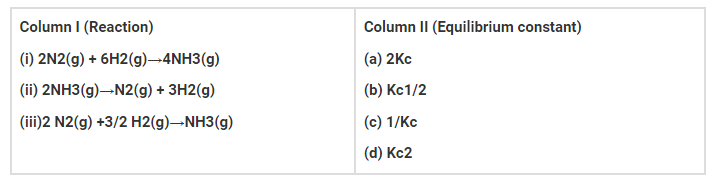# For the reaction : N2 (g) + 3H2(g) → 2NH3(g)`
Question:

For the reaction : N2 (g) + 3H2(g) → 2NH3(g)

Equilibrium constant Kc= [NH3]2/[N2][H2]3

Some reactions are written below in Column I and their equilibrium constants

in terms of Kc

are written in Column II. Match the following reactions with the

corresponding equilibrium constantSolution:

(i) is d

(ii) is c

(iii) is b